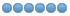# How to Control XYZ With a analog Joystick (model: r400b-m4)

• Michael
• Offline
• Platinum Member
•19 Dec 2021 23:16 - 19 Dec 2021 23:54 #229524 by Michael
I was assuming each direction of the axis had a separate pot. All I had to go off was the picture. If its one pot per axis its pretty simple change to the code above.

#net jog-x-analog  =>  halui.axis.x.analog
net jog-x-analog  <=  scale.xjog.out

setp scale.xjog.gain .083
net xjog-scale <= scale.x.out
net xjog-scale => scale.xjog.out

setp scale.x.offset -12
setp scale.x.gain 1
net x-scale  =>  scale.x.in

The logic for the scale component would be:
out = in * gain + offset
First scale will split the  0 to 24v into -12v to 12v
-12 = 0v * 1 - 12   (full left)
-9 = 3v * 1 - 12   (3/4 left)
0 = 12v * 1 - 12   (no movement)
3 = 15v * 1 - 12   (1/4 right)
12 = 24v * 1 - 12   (full right)

Then you need to bring the scale into the range of -1 to +1 for the "halui.axis.x.analog" signal using the results of the first scale component
-.996 = -12 * .083   (full left)
-.747 = -9 * .083   (3/4 left)
0 = 0 * .083   (no movement)
.249 = 3 * .083   (1/4 right)
.996 = 12 * .083   (full right)

edit: You may be able to work it through a single scale component but would need to verify how it does the math with gain and offset.
Last edit: 19 Dec 2021 23:54 by Michael.